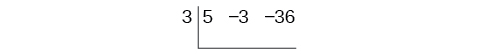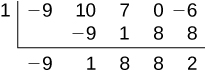# 5.4 Dividing polynomials  (Page 3/6)

 Page 3 / 6

## Using synthetic division to divide a second-degree polynomial

Use synthetic division to divide $\text{\hspace{0.17em}}5{x}^{2}-3x-36\text{\hspace{0.17em}}$ by $\text{\hspace{0.17em}}x-3.\text{\hspace{0.17em}}$

Begin by setting up the synthetic division. Write $\text{\hspace{0.17em}}k\text{\hspace{0.17em}}$ and the coefficients.Bring down the lead coefficient. Multiply the lead coefficient by $\text{\hspace{0.17em}}k.\text{\hspace{0.17em}}$Continue by adding the numbers in the second column. Multiply the resulting number by $\text{\hspace{0.17em}}k.\text{\hspace{0.17em}}$ Write the result in the next column. Then add the numbers in the third column.The result is $\text{\hspace{0.17em}}5x+12.\text{\hspace{0.17em}}$ The remainder is 0. So $\text{\hspace{0.17em}}x-3\text{\hspace{0.17em}}$ is a factor of the original polynomial.

## Using synthetic division to divide a third-degree polynomial

Use synthetic division to divide $\text{\hspace{0.17em}}4{x}^{3}+10{x}^{2}-6x-20\text{\hspace{0.17em}}$ by $\text{\hspace{0.17em}}x+2.\text{\hspace{0.17em}}$

The binomial divisor is $\text{\hspace{0.17em}}x+2\text{\hspace{0.17em}}$ so $\text{\hspace{0.17em}}k=-2.\text{\hspace{0.17em}}$ Add each column, multiply the result by –2, and repeat until the last column is reached.The result is $\text{\hspace{0.17em}}4{x}^{2}+2x-10.\text{\hspace{0.17em}}$ The remainder is 0. Thus, $\text{\hspace{0.17em}}x+2\text{\hspace{0.17em}}$ is a factor of $\text{\hspace{0.17em}}4{x}^{3}+10{x}^{2}-6x-20.\text{\hspace{0.17em}}$

## Using synthetic division to divide a fourth-degree polynomial

Use synthetic division to divide $\text{\hspace{0.17em}}-9{x}^{4}+10{x}^{3}+7{x}^{2}-6\text{\hspace{0.17em}}$ by $\text{\hspace{0.17em}}x-1.\text{\hspace{0.17em}}$

Notice there is no x -term. We will use a zero as the coefficient for that term.The result is $\text{\hspace{0.17em}}-9{x}^{3}+{x}^{2}+8x+8+\frac{2}{x-1}.$

Use synthetic division to divide $\text{\hspace{0.17em}}3{x}^{4}+18{x}^{3}-3x+40\text{\hspace{0.17em}}$ by $\text{\hspace{0.17em}}x+7.$

$3{x}^{3}-3{x}^{2}+21x-150+\frac{1,090}{x+7}$

## Using polynomial division to solve application problems

Polynomial division can be used to solve a variety of application problems involving expressions for area and volume. We looked at an application at the beginning of this section. Now we will solve that problem in the following example.

## Using polynomial division in an application problem

The volume of a rectangular solid is given by the polynomial $\text{\hspace{0.17em}}3{x}^{4}-3{x}^{3}-33{x}^{2}+54x.\text{\hspace{0.17em}}$ The length of the solid is given by $\text{\hspace{0.17em}}3x\text{\hspace{0.17em}}$ and the width is given by $\text{\hspace{0.17em}}x-2.\text{\hspace{0.17em}}$ Find the height, $\text{\hspace{0.17em}}t,$ of the solid.

There are a few ways to approach this problem. We need to divide the expression for the volume of the solid by the expressions for the length and width. Let us create a sketch as in [link] .

We can now write an equation by substituting the known values into the formula for the volume of a rectangular solid.

$\begin{array}{ccc}\hfill V& =& l\cdot w\cdot h\hfill \\ \hfill 3{x}^{4}-3{x}^{3}-33{x}^{2}+54x& =& 3x\cdot \left(x-2\right)\cdot h\hfill \end{array}$

To solve for $\text{\hspace{0.17em}}h,\text{\hspace{0.17em}}$ first divide both sides by $\text{\hspace{0.17em}}3x.$

$\begin{array}{ccc}\hfill \frac{3x\cdot \left(x-2\right)\cdot h}{3x}& =& \frac{3{x}^{4}-3{x}^{3}-33{x}^{2}+54x}{3x}\hfill \\ \hfill \left(x-2\right)h& =& {x}^{3}-{x}^{2}-11x+18\hfill \end{array}$

Now solve for $\text{\hspace{0.17em}}h\text{\hspace{0.17em}}$ using synthetic division.

$h=\frac{{x}^{3}-{x}^{2}-11x+18}{x-2}$The quotient is $\text{\hspace{0.17em}}{x}^{2}+x-9\text{\hspace{0.17em}}$ and the remainder is 0. The height of the solid is $\text{\hspace{0.17em}}{x}^{2}+x-9.$

The area of a rectangle is given by $\text{\hspace{0.17em}}3{x}^{3}+14{x}^{2}-23x+6.\text{\hspace{0.17em}}$ The width of the rectangle is given by $\text{\hspace{0.17em}}x+6.\text{\hspace{0.17em}}$ Find an expression for the length of the rectangle.

$3{x}^{2}-4x+1$

Access these online resources for additional instruction and practice with polynomial division.

## Key equations

 Division Algorithm

## Key concepts

• Polynomial long division can be used to divide a polynomial by any polynomial with equal or lower degree. See [link] and [link] .
• The Division Algorithm tells us that a polynomial dividend can be written as the product of the divisor and the quotient added to the remainder.
• Synthetic division is a shortcut that can be used to divide a polynomial by a binomial in the form $\text{\hspace{0.17em}}x-k.\text{\hspace{0.17em}}$ See [link] , [link] , and [link] .
• Polynomial division can be used to solve application problems, including area and volume. See [link] .

how do I set up the problem?
what is a solution set?
Harshika
find the subring of gaussian integers?
Rofiqul
hello, I am happy to help!
Abdullahi
hi mam
Mark
find the value of 2x=32
divide by 2 on each side of the equal sign to solve for x
corri
X=16
Michael
Want to review on complex number 1.What are complex number 2.How to solve complex number problems.
Beyan
yes i wantt to review
Mark
use the y -intercept and slope to sketch the graph of the equation y=6x
how do we prove the quadratic formular
Darius
hello, if you have a question about Algebra 2. I may be able to help. I am an Algebra 2 Teacher
thank you help me with how to prove the quadratic equation
Seidu
may God blessed u for that. Please I want u to help me in sets.
Opoku
what is math number
4
Trista
x-2y+3z=-3 2x-y+z=7 -x+3y-z=6
can you teacch how to solve that🙏
Mark
Solve for the first variable in one of the equations, then substitute the result into the other equation. Point For: (6111,4111,−411)(6111,4111,-411) Equation Form: x=6111,y=4111,z=−411x=6111,y=4111,z=-411
Brenna
(61/11,41/11,−4/11)
Brenna
x=61/11 y=41/11 z=−4/11 x=61/11 y=41/11 z=-4/11
Brenna
Need help solving this problem (2/7)^-2
x+2y-z=7
Sidiki
what is the coefficient of -4×
-1
Shedrak
the operation * is x * y =x + y/ 1+(x × y) show if the operation is commutative if x × y is not equal to -1
An investment account was opened with an initial deposit of \$9,600 and earns 7.4% interest, compounded continuously. How much will the account be worth after 15 years?
lim x to infinity e^1-e^-1/log(1+x)
given eccentricity and a point find the equiationByByBy Briana KnowltonBy Mahee BooBy Darlene PaliswatBy Rohini AjayBy OpenStaxBy Maureen MillerBy Eric CrawfordBy OpenStaxByBy Christine Zeelie